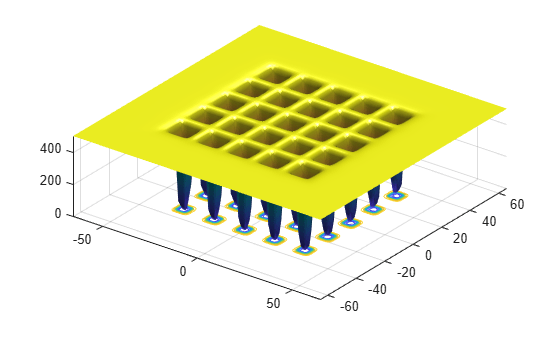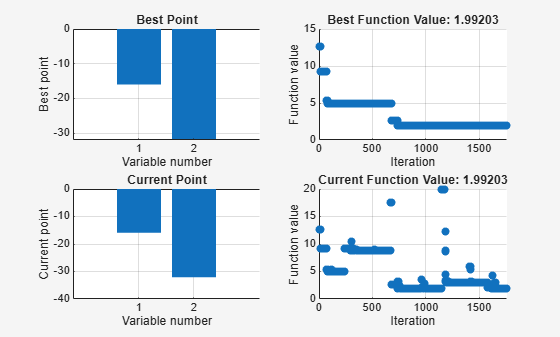simulannealbnd

Find minimum of function using simulated annealing algorithm

Description

example

x = simulannealbnd(fun,x0) finds a local minimum, x, to the function handle fun that computes the values of the objective function. x0 is an initial point for the simulated annealing algorithm, a real vector.

Note

Passing Extra Parameters explains how to pass extra parameters to the objective function, if necessary.

example

x = simulannealbnd(fun,x0,lb,ub) defines a set of lower and upper bounds on the design variables in x, so that the solution is always in the range lb  x  ub. If x(i) is unbounded below, set lb(i) = -Inf, and if x(i) is unbounded above, set ub(i) = Inf.

example

x = simulannealbnd(fun,x0,lb,ub,options) minimizes with the optimization options specified in options. Create options using optimoptions. If no bounds exist, set lb = [] and/or ub = [].

x = simulannealbnd(problem) finds the minimum for problem, a structure described in problem.

[x,fval] = simulannealbnd(___), for any syntax, returns the value of the objective function fun at the solution x.

example

[x,fval,exitflag,output] = simulannealbnd(___) additionally returns a value exitflag that describes the exit condition of simulannealbnd, and a structure output with information about the optimization process.

Examples

collapse all

Minimize De Jong's fifth function, a two-dimensional function with many local minima.

Plot De Jong's fifth function.

dejong5fcnMinimize De Jong's fifth function using simulannealbnd starting from the point [0,0].

fun = @dejong5fcn;
x0 = [0 0];
x = simulannealbnd(fun,x0)
Optimization terminated: change in best function value less than options.FunctionTolerance.
x = 1×2

-32.0285   -0.1280

The simulannealbnd algorithm uses the MATLAB® random number stream, so you might obtain a different result.

Minimize De Jong’s fifth function within a bounded region.

Plot De Jong's fifth function.

dejong5fcnStart simulannealbnd starting at the point [0,0], and set lower bounds of -64 and upper bounds of 64 on each component.

fun = @dejong5fcn;
x0 = [0 0];
lb = [-64 -64];
ub = [64 64];
x = simulannealbnd(fun,x0,lb,ub)
Optimization terminated: change in best function value less than options.FunctionTolerance.
x = 1×2

-15.9790  -31.9593

The simulannealbnd algorithm uses the MATLAB® random number stream, so you might obtain a different result.

Observe the progress of simulannealbnd by setting options to use some plot functions.

Set simulated annealing options to use several plot functions.

options = optimoptions('simulannealbnd','PlotFcns',...
{@saplotbestx,@saplotbestf,@saplotx,@saplotf});

Start simulannealbnd starting at the point [0,0], and set lower bounds of -64 and upper bounds of 64 on each component.

rng default % For reproducibility
fun = @dejong5fcn;
x0 = [0,0];
lb = [-64,-64];
ub = [64,64];
x = simulannealbnd(fun,x0,lb,ub,options)Optimization terminated: change in best function value less than options.FunctionTolerance.
x = 1×2

-15.9790  -31.9593

Obtain all the outputs of a simulated annealing minimization.

Plot De Jong's fifth function.

dejong5fcnStart simulannealbnd starting at the point [0,0], and set lower bounds of -64 and upper bounds of 64 on each component.

fun = @dejong5fcn;
x0 = [0,0];
lb = [-64,-64];
ub = [64,64];
[x,fval,exitflag,output] = simulannealbnd(fun,x0,lb,ub)
Optimization terminated: change in best function value less than options.FunctionTolerance.
x = 1×2

-15.9790  -31.9593

fval = 1.9920
exitflag = 1
output = struct with fields:
iterations: 1762
funccount: 1779
message: 'Optimization terminated: change in best function value less than options.FunctionTolerance.'
rngstate: [1x1 struct]
problemtype: 'boundconstraints'
temperature: [2x1 double]
totaltime: 0.9710

The simulannealbnd algorithm uses the MATLAB® random number stream, so you might obtain a different result.

Input Arguments

collapse all

Function to be minimized, specified as a function handle or function name.fun is a function that accepts a vector x and returns a real scalar f, the objective function evaluated at x.

fun can be specified as a function handle for a file:

x = simulannealbnd(@myfun,x0)

where myfun is a MATLAB® function such as

function f = myfun(x)
f = ...            % Compute function value at x

fun can also be a function handle for an anonymous function:

x = simulannealbnd(@(x)norm(x)^2,x0,lb,ub);

Example: fun = @(x)sin(x(1))*cos(x(2))

Data Types: char | function_handle | string

Initial point, specified as a real vector. simulannealbnd uses the number of elements in x0 to determine the number of variables that fun accepts.

Example: x0 = [1,2,3,4]

Data Types: double

Lower bounds, specified as a real vector or real array. If the number of elements in x0 is equal to that of lb, then lb specifies that

x(i) >= lb(i) for all i.

If numel(lb) < numel(x0), then lb specifies that

x(i) >= lb(i) for 1 <= i <= numel(lb).

In this case, solvers issue a warning.

Example: To specify that all control variables are positive, lb = zeros(size(x0))

Data Types: double

Upper bounds, specified as a real vector or real array. If the number of elements in x0 is equal to that of ub, then ub specifies that

x(i) <= ub(i) for all i.

If numel(ub) < numel(x0), then ub specifies that

x(i) <= ub(i) for 1 <= i <= numel(ub).

In this case, solvers issue a warning.

Example: To specify that all control variables are less than one, ub = ones(size(x0))

Data Types: double

Optimization options, specified as an object returned by optimoptions or a structure. For details, see Simulated Annealing Options.

optimoptions hides the options listed in italics; see Options that optimoptions Hides.

{} denotes the default value. See option details in Simulated Annealing Options.

OptionDescriptionValues

AcceptanceFcn

Function the algorithm uses to determine if a new point is accepted. Specify as 'acceptancesa' or a function handle.

Function handle | {'acceptancesa'}

AnnealingFcn

Function the algorithm uses to generate new points. Specify as a name of a built-in annealing function or a function handle.

Function handle | function name | 'annealingboltz' | {'annealingfast'}

DataType

Type of decision variable

'custom' | {'double'}

Display

Level of display

'off' | 'iter' | 'diagnose' | {'final'}

DisplayInterval

Interval for iterative display

Positive integer | {10}

FunctionTolerance

Termination tolerance on function value

For an options structure, use TolFun.

Positive scalar | {1e-6}

HybridFcn

Automatically run HybridFcn (another optimization function) during or at the end of iterations of the solver. Specify as a name or a function handle.

'fminsearch' | 'patternsearch' | 'fminunc' | 'fmincon' | {[]}

or

1-by-2 cell array | {@solver, hybridoptions}, where solver = fminsearch, patternsearch, fminunc, or fmincon {[]}

HybridInterval

Interval (if not 'end' or 'never') at which HybridFcn is called

Positive integer | 'never' | {'end'}

InitialTemperature

Initial value of temperature

Positive scalar | positive vector | {100}

MaxFunctionEvaluations

Maximum number of objective function evaluations allowed

For an options structure, use MaxFunEvals.

Positive integer | {3000*numberOfVariables}

MaxIterations

Maximum number of iterations allowed

For an options structure, use MaxIter.

Positive integer | {Inf}

MaxStallIterations

Number of iterations over which average change in fitness function value at current point is less than options.FunctionTolerance

For an options structure, use StallIterLimit.

Positive integer | {500*numberOfVariables}

MaxTime

The algorithm stops after running for MaxTime seconds

For an options structure, use TimeLimit.

Positive scalar | {Inf}

ObjectiveLimit

Minimum objective function value desired

Scalar | {-Inf}

OutputFcn

Function(s) get(s) iterative data and can change options at run time

For an options structure, use OutputFcns.

Function handle | cell array of function handles | {[]}

PlotFcn

Plot function(s) called during iterations

For an options structure, use PlotFcns.

Function handle | built-in plot function name | cell array of function handles | cell array of built-in plot function names | 'saplotbestf' | 'saplotbestx' | 'saplotf' | 'saplotstopping' | 'saplottemperature' | {[]}

PlotInterval

Plot functions are called at every interval

Positive integer | {1}

ReannealInterval

Reannealing interval

Positive integer | {100}

TemperatureFcn

Function used to update temperature schedule

Function handle | built-in temperature function name | 'temperatureboltz' | 'temperaturefast' | {'temperatureexp'}

Example: options = optimoptions(@simulannealbnd,'MaxIterations',150)

Data Types: struct

Problem structure, specified as a structure with the following fields:

• objective — Objective function

• x0 — Starting point

• lb — Lower bound for x

• ub — Upper bound for x

• solver'simulannealbnd'

• options — Options created with optimoptions or an options structure

• rngstate — Optional field to reset the state of the random number generator

Note

problem must have all the fields as specified above.

Data Types: struct

Output Arguments

collapse all

Solution, returned as a real vector. The size of x is the same as the size of x0. Typically, x is a local solution to the problem when exitflag is positive.

Objective function value at the solution, returned as a real number. Generally, fval = fun(x).

Reason simulannealbnd stopped, returned as an integer.

Exit FlagMeaning
1

Average change in the value of the objective function over options.MaxStallIterations iterations is less than options.FunctionTolerance.

5

Objective function value is less than options.ObjectiveLimit.

0

Maximum number of function evaluations or iterations reached.

-1

Optimization terminated by an output function or plot function.

-2

No feasible point found.

-5

Time limit exceeded.

Information about the optimization process, returned as a structure with fields:

• problemtype — Type of problem: unconstrained or bound constrained.

• iterations — The number of iterations computed.

• funccount — The number of evaluations of the objective function.

• message — The reason the algorithm terminated.

• temperature — Temperature when the solver terminated.

• totaltime — Total time for the solver to run.

• rngstate — State of the MATLAB random number generator, just before the algorithm started. You can use the values in rngstate to reproduce the output of simulannealbnd. See Reproduce Your Results.

Alternative Functionality

App

The Optimize Live Editor task provides a visual interface for simulannealbnd.

Introduced in R2007a

Global Optimization Toolbox DocumentationGet trial now